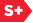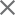# Improved surface measurements of large spaceborne mirrors

A new pixel-based absolute test method is used to determine the zero-gravity surface shape of a target mirror under normal gravity conditions and to remove interferometric errors.
17 June 2016
Hyug-Gyo Rhee, Hagyong Kihm, Ho-Soon Yang and Yun-Woo Lee

High-accuracy interferometry methods are required to measure the surface shapes of large mirrors that are used in spaceborne telescopes. Indeed, it is often necessary for such mirrors to be tested and fabricated to a more accurate standard than the reference optics with which they are compared. Furthermore, the precise measurements (i.e., with nanometer-scale accuracy) for these large mirrors must be made in gravity-free (weightless) conditions. To address these requirements, an absolute approach therefore needs to be adopted so that the surface shape of the target mirror can be separated from errors caused by the interferometer and the effect of gravity.In several previous studies,1–16 an absolute test has been performed to distinguish between inaccuracies in the interferometer readings and the true surface shape of the target mirror. These previous studies, however, had two main problems. First, it was assumed that no azimuthal position error arises during rotation of the mirror. When the mirror is small enough and an ultraprecision rotator is used, the azimuthal position error is indeed negligible. For large mirrors, however, it is physically difficult to achieve no azimuthal position error. The second, and more serious, problem is that only three rotations (120° spacing) are available for a spaceborne mirror because they generally have three bosses at the rim for flexure mounting. With the earlier methodologies it is not possible to successfully remove the third angular harmonic error components (which is where the gravity effect most strongly appears). Moreover, in most spaceborne mirrors, complicated pocket patterns are used to achieve the required optical performance with a minimum mass. These patterns, however, have an influence on the front surface of the mirrors. For a mirror with a diameter of 1m, the number of pockets can be as high as 100. This causes the ‘quilting effect’ to occur at high spatial frequencies, which cannot be represented by low- and middle-order Zernike polynomials.

We have been motivated by all these issues to introduce a new pixel-based absolute test method for measuring the surface shape of large spaceborne mirrors. With our approach we adopt an optimization technique to determine the zero-gravity surface shape of a target mirror under normal gravity conditions. We are also able to remove the systematic errors caused by the interferometer.17

To demonstrate our proposed method, we have fabricated a lightweight primary mirror system that has a diameter of 1m (with 96 pockets on its back side) and three bosses at its rim for flexure mounting. This mirror is a candidate for South Korea's next satellite telescope. To measure the shape of the mirror we used a vibration-desensitized interferometer that has a computer-generated hologram as a null corrector (see Figure 1). This measurement inevitably included both the gravity effect and the systematic errors associated with the interferometer, and can be expressed according to Equation 1:where W is the shape term, and where I, G, and S denote the systematic error of the interferometer, the gravity effect, and the surface shape of the mirror, respectively, at a specific position with polar coordinates (r, θ). The subscripts denote the rotation index (which can vary from 0 to 2, where 0 is no rotation). After the first rotation (in the counterclockwise direction) of our measurement, the rotated data can thus be described as:From Equation 2 we can appreciate that a rotated (by angle α1) surface shape is related to the original surface shape and its conjugate form,. In an intermediate step, we substitute Equation 1 into Equation 2 to define the difference in the surface shape. Then, in the last part of our methodology, the actual measured value of the surface shape is used to define the merit function of the mirror. It is this function that we optimize to determine the ‘true’ surface shape at each pixel point.Figure 1. (a) Photograph of the polished surface of the lightweight 1m-diameter mirror with 96 pockets, after flexure bonding. (b) The surface shape measurement setup, which takes place after the surface has been coated with silver.Figure 2. (A) Photograph illustrating the rotation index for each boss of the mirror (i.e., b1, b2, and b3). The mirror surface shape measurement results obtained at the three different rotation positions are shown in (B), (C), and (D). The measurements range from - 149.1 (blue) to 121.8nm (red). (E) The ‘true’ mirror shape, as calculated from the merit function. (F) The experimentally obtained errors, caused by both the interferometer and the gravity effect. (G) The gravity effect errors, as calculated by finite element analysis (FEA).

We show typical surface measurement data at three different rotation positions in Figure 2(B) to (D). We averaged three sets of measurement data at each position so that we reduced the random noise, and we used these averages to calculate the merit function. The final ‘true’ mirror shape result is shown in Figure 2(E). From this data, we also estimated that the zero-gravity surface shape had a peak-to-valley (PV) value of 59.4nm and a root mean square (RMS) of 8.4nm. We compared this experimental result with a finite element analysis computer simulation of the mirror surface. We thus predicted—see Figure 2(G)—that the gravity effect had PV and RMS values of 50.3 and 9.1nm, respectively, and found some similarity between the experimental and simulation data sets. We also determined, by using the rule of square summation, that the interferometer error had a PV value of about 41nm and an RMS of about 7.9nm.

In another demonstration of our methodology, we tested an ‘upside-down’ measurement case (see Figure 3). In this test, we made shape measurements at three rotation positions to check how they (i.e., with gravity in the opposite direction) varied from our original measurements. We found that the true surface shape result from these measurements—see Figure 3(E)—was similar to a version of the results shown in Figure 2(E) that were rotated by 180°. This was a reasonable finding because the mirror had indeed been rotated by this angle.Figure 3. (A) Photograph illustrating the upside-down surface measurement case (i.e., where the mirror has been rotated by 180° compared with the case illustrated in Figure 2). The experimental surface shape measurements for the mirror are shown for three different rotation positions in (B), (C), and (D). The measurements range from –149.1 (blue) to 121.8nm (red). (E) The true surface mirror shape (from the merit function). (F) The experimental errors from the interferometer and the gravity effect. (G) The gravity effect errors calculated by FEA.

In summary, we have experimentally verified a new pixel-based method for absolute measurements of the surface shape of large, lightweight mirrors. In our optimization approach we can calculate the zero-gravity shape of the target mirror, but under normal gravity conditions. Our technique is therefore suitable for surface measurements of mirrors for spaceborne telescopes. With our method, we also remove systematic errors that arise from the interferometer. In our future work we are planning to apply our technique for the testing of the Giant Magellan Telescope secondary mirrors.

Hyug-Gyo Rhee, Hagyong Kihm, Ho-Soon Yang, Yun-Woo Lee
Korea Research Institute of Standards and Science (KRISS)
Daejeon, Republic of Korea

Hyug-Gyo Rhee received his MS and PhD in mechanical engineering from KRISS in 1999 and 2003, respectively. He then worked for the National Institute of Standards and Technology as an optical scientist until he joined KRISS in 2005. His research interests are space and airborne optics, optomechatronics, interferometry, and freeform optics.

References:
1. A. E. Jensen, Absolute calibration method for laser Twyman-Green wavefront testing interferometers, Proc. Fall Opt. Soc. Am. Mtg., p. ThG19, 1973.
2. K. L. Shu, Ray-trace analysis and data reduction methods for the Ritchey-Common test, Appl. Opt. 22, p. 1879-1886, 1983.
3. B. S. Fritz, Absolute calibration of an optical flat, Opt. Eng. 23, p. 234379, 1984. doi:10.1117/12.7973304
4. K.-E. Elssner, R. Burow, J. Grzanna, R. Spolaczyk, Absolute sphericity measurement, Appl. Opt. 28, p. 4649-4661, 1989.
5. G. Schulz, J. Grzanna, Absolute flatness testing by the rotation method with optimal measuring-error compensation, Appl. Opt. 31, p. 3767-3780, 1992.
6. P. Hariharan, Interferometric testing of optical surfaces: absolute measurements of flatness, Opt. Eng. 36, p. 2478-2481, 1997. doi:10.1117/1.601474
7. V. Greco, R. Tronconi, C. Del Vecchio, M. Trivi, G. Molesini, Absolute measurement of planarity with Fritz's method: uncertainty evaluation, Appl. Opt. 38, p. 2018-2027, 1999.
8. P. E. Murphy, T. G. Brown, D. T. Moore, Interference imaging for aspheric surface testing, Appl. Opt. 39, p. 2122-2129, 2000.
9. K. R. Freischlad, Absolute interferometric testing based on reconstruction of rotational shear, Appl. Opt. 40, p. 1637-1648, 2001.
10. S. Reichelt, C. Pruss, H. J. Tiziani, Absolute interferometric test of aspheres by use of twin computer-generated holograms, Appl. Opt. 42, p. 4468-4479, 2003.
11. C. Ai, J. C. Wyant, Absolute testing of flats by using even and odd functions, Appl. Opt. 32, p. 4698-4705, 1993.
12. C. J. Evans, R. N. Kestner, Test optics error removal, Appl. Opt. 35, p. 1015-1021, 1996.
13. W. T. Estler, C. J. Evans, L. Z. Shao, Uncertainty estimation for multiposition form error metrology, Precis. Eng. 21, p. 72-82, 1997.
14. R. E. Parks, L. Shao, C. J. Evans, Pixel-based absolute topography test for three flats, Appl. Opt. 37, p. 5951-5956, 1998.
15. U. Griesmann, Three-flat test solutions based on simple mirror symmetry, Appl. Opt. 45, p. 5856-5865, 2006.
16. E. E. Bloemhof, J. C. Lam, V. A. Feria, Z. Chang, Extracting the zero-gravity surface figure of a mirror through multiple clockings in a flightlike hexapod mount, Appl. Opt. 48, p. 4239-4245, 2009.
17. H.-G. Rhee, H. Kihm, H.-S. Yang, Y.-S. Ghim, Y.-W. Lee, J. Lee, Pixel-based absolute test of a 1-m lightweight mirror for a space telescope, J. Korean Phys. Soc. 65, p. 1385-1389, 2014.
Recent News